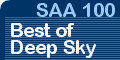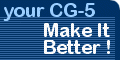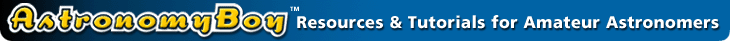Search AstronomyBoy with Google:Getting StartedCG-5/LX70 Mount FixesSolar Eclipse — 2017SAA 100 Deep Sky ListConstellation PortraitsBarn Door TrackerComet Hale-BoppHomemade EyepiecesEQ Mount TutorialMillennium RantWho Is Astronomy Boy?Contact Astronomy BoyHomeEyepiece Calculations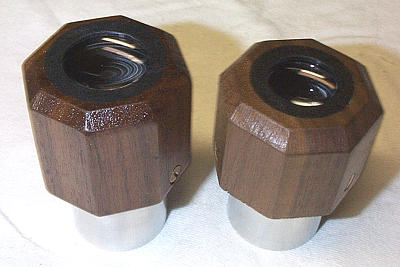Eyepiece Focal Length Calculators
The calculators below perform a rough determination of the Effective Focal Length (EFL) of simple 2- and 3-element eyepieces. You need only specify the focal length of the individual elements and the spacing between the elements.

The formulas employed by these calculators are approximations. I have found them to be reasonably accurate in predicting the focal lengths of my homemade eyepieces.

Definitions
The Effective Focal Length (EFL) is what we commonly refer to as the focal length of an eyepiece. It takes into account the focal lengths of the individual lens elements and the spacing between those elements.

An element can be a simple lens consisting of one piece of glass, a doublet or achromat (two pieces of glass cemented together), or even a triplet (three pieces of glass cemented together). In this discussion, any of these is considered to be one element. Here are some examples.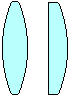Simple Lenses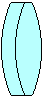Achromat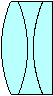Triplet
The spacing is the distance between the principal planes of two adjacent elements. In the calculators below, it is assumed that the principal plane of a simple lens lies in the center of the lens. For an achromat, it is assumed to be where the two pieces of glass are joined in the center of the lens. For a triplet, the principal plane is assume to be in the center of the middle piece of glass.

In a multi-element eyepiece, the element nearest your eye is called the eye lens. The element at the opposite end of the eyepiece, closest to the objective of the telescope, is the field lens. If there is a third element in between these two, it is simply referred to as the middle lens.

Practical Considerations
Most simple eyepiece designs (Kellners, Symmetricals, Plössls, even Erfles) specify that the elements be closely spaced – nearly touching. For the lenses commonly used to construct 1.25-inch eyepieces, this puts the principal planes on the order of 4mm to 10mm apart. Modest changes in spacing have little effect on the EFL.

Increasing the distance between elements will increase the EFL but may introduce other undesireable characteristics, such as a narrower field of view. I've found that simple eyepieces with closely spaced lenses usually have a field of view of 45° to 50°.

To maximize eye relief, arrange the elements so that the lens with the longest focal length is the field lens and the one with the shortest focal length is the eye lens.

Limitations
These calculators tell you nothing about the field of view or eye relief of an eyepiece. They are useful only for calculating the Effective Focal Length, given the focal length and spacing of the lens elements.

The calculators below are also available as an Excel spreadsheet.Click to download.

2-Element Focal Length Calculator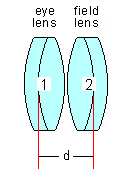Enter the focal lengths of the lenses (f1 and f2), and the spacing (d) between the principal planes of the elements. Then click Calculate EFL to perform the calculation. The lens types shown in the diagram are for illustration only; many different arrangements are possible. The calculator does not care what type of lens is used in each position. f1 f2 d EFL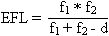3-Element Focal Length Calculator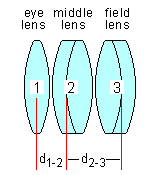Enter the focal lengths of the lenses (f1, f2 and f3), and the spacing (d1-2 and d2-3) between the principal planes of adjacent elements. Then click Calculate EFL to perform the calculation. The lens types shown in the diagram are for illustration only; many different arrangements are possible. The calculator does not care what type of lens is used in each position.
 f1 f2 d1-2 f3 d2-3 EFL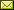Web site by DeTray Associateswww.DeTray.com Privacy Policy